July 7, 2007

Mathematical Paris

Posted by John BaezI’m in Paris from July 1st to 19th. Just for fun, I’ve started taking pictures of streets named after mathematicians. Can you help me find more of these streets?

The day after I arrived was the day that exam scores were posted for all schools in France — from elementary schools to the Sorbonne. Students and some parents lined up, anxiously awaiting the results: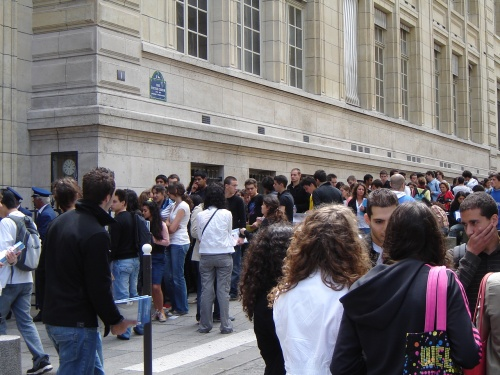Perhaps it was no coincidence that many cars parked next to the Sorbonne had these fliers placed on their windshieds: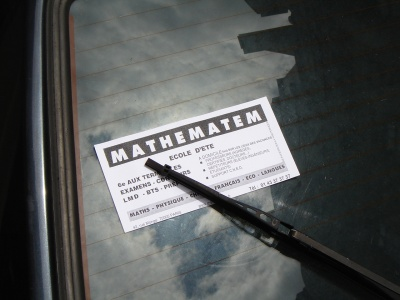Indeed, Paris is a city of mathematicians. Just for fun, I began taking photographs of streets named after mathematicians… or more precisely, of the street signs. Today I got two: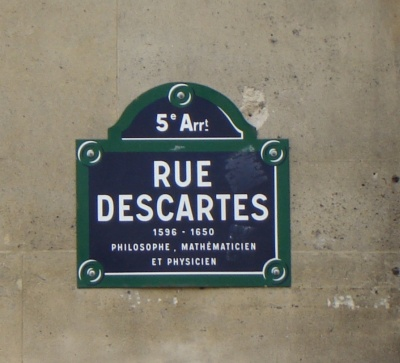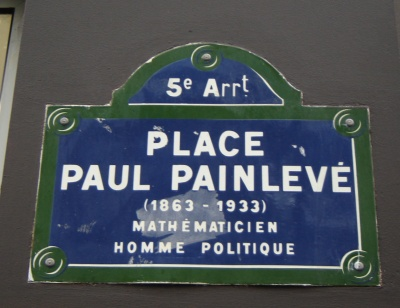Descartes you surely know. Paul Painlevé may be best known as a politician in France, but mathematicians know him for his work on differential equations.

I need to get Rue Monge and Rue Laplace! Do you know more streets in Paris named after mathematicians — especially near the 5th and 6th arrondisements?

Posted at July 7, 2007 9:51 AM UTC

TrackBack URL for this Entry:   https://golem.ph.utexas.edu/cgi-bin/MT-3.0/dxy-tb.fcgi/1350

Re: Mathematical Paris

There’s the Rue Henri Poincaré, but it’s out in the $20^{th}$.

Posted by: David Corfield on July 7, 2007 10:22 AM | Permalink | Reply to this

Re: Mathematical Paris

South of Étoile there are Rue Newton, Rue Euler, Rue Galilée and Rue Kepler.

Posted by: David Corfield on July 7, 2007 10:31 AM | Permalink | Reply to this

Re: Mathematical Paris

If you go to Marseille you will find the rue Frégier. He did a theorem about a point associated to conics in the projective space in early ninetenth century. It is funny because I did receive a mail by a mathematician of the Russian Academy of sciences who asked (he is writing an encyclopedic dictionnary)me if these were my results (!)

Posted by: frégier on July 7, 2007 12:09 PM | Permalink | Reply to this

Re: Mathematical Paris

Posted by: Pioneer1 on July 7, 2007 1:41 PM | Permalink | Reply to this

Re: Mathematical Paris

Cool! Thanks! I’m glad it doesn’t have photos of all the streets — it might have reduced my will to carry out this project.Over a hundred streets named after mathematicians! I guess I’ll only do the nearby ones.

Posted by: John Baez on July 7, 2007 9:49 PM | Permalink | Reply to this

Re: Mathematical Paris

Talk about convergent math blogging; check out the discussion yesterday at http://sbseminar.wordpress.com/2007/07/06/56/

Posted by: Scott Morrison on July 7, 2007 2:17 PM | Permalink | PGP Sig | Reply to this

Re: Mathematical Paris

Tu peux aller au cimetière du Père Lachaise.

Il y a les tombes de Chasles, Fourier, Fresnel, Monge (transféré au Panthéon ).

Posted by: beverycool on July 7, 2007 2:54 PM | Permalink | Reply to this

Re: Mathematical Paris

Il y a aussi Lagrange au Panthéon et Descartes est à l’église de Saint Germain des Prés.

Bonne visite.

Posted by: beverycool on July 7, 2007 3:25 PM | Permalink | Reply to this

Re: Mathematical Paris

Posted by: ReiVaX18 on July 7, 2007 6:35 PM | Permalink | Reply to this

Mode of Street Names; Re: Mathematical Paris

Many “Post Street” instances. But are any based on Emil Post? I am NOT that Post. I keep telling you that. So stop sending me those unpaid bills.

Nor am I related to the E. J Post of “Formal structure of electromagnetics: General covariance and electromagnetics” and “Can microphysical structure be probed by period integrals?”
Phys. Rev. D 25, 3223 - 3229 (1982)
[Issue 12-13; June 1982]
[E. J. Post
8634-3 Pershing Drive, Playa del Rey, California 90291]

But due to the co-California addresses, we HAVE gotten mail intended for each other.

Is there a Prime Street? Is it infinitely long?

There are MANY “Euclid Avenues” in the USA, as geometry actually used to be taught in ALL American schools, and used by all surveyors.

The related question:

What is the MOST common street name in the USA?

This is actually mathematical and logical…

Posted by: Jonathan Vos Post on July 7, 2007 6:44 PM | Permalink | Reply to this

Re: Mode of Street Names; Re: Mathematical Paris

Main?

Posted by: jim stasheff on July 12, 2007 1:56 AM | Permalink | Reply to this

Re: Mode of Street Names; Re: Mathematical Paris

I guessed “Main” too, but then rejected it as being too obvious. However, “Main” is virtually a synonym of another obvious candidate, and that very fact is a clue…

Posted by: Todd Trimble on July 13, 2007 12:59 PM | Permalink | Reply to this

Re: Mathematical Paris

In the morning Lisa and I went on a long walk to the west end of Saint-Germaine, and then back east… and I managed to snap a picture of Rue Lagrange: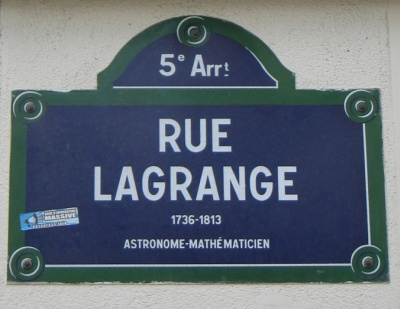In the afternoon we went on another walk, up to the Right Bank, and on the way back I got a shot of Rue Monge: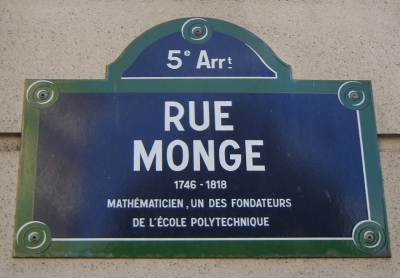Posted by: John Baez on July 7, 2007 9:36 PM | Permalink | Reply to this

Re: Mathematical Paris

Look for “Rue Raymond Poincaré”, Paris 16e.

There is also “Rue Euler”, Paris 8e.

Posted by: Pedro on July 8, 2007 3:10 PM | Permalink | Reply to this

Re: Mathematical Paris

Raymond was a politician, prime minister and President. It’s Henri you want, often described as his cousin, whose only manages a street mention in the $20^{th}$.

Posted by: David Corfield on July 9, 2007 9:45 AM | Permalink | Reply to this

Re: Mathematical Paris

Today I bagged Rue Laplace, just north of the Pantheon: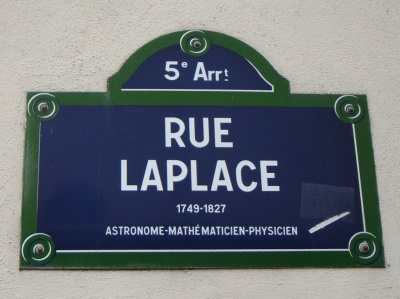It looks like Rue Malus and Rue Malebranche are the only ones left in the 5th arrondisement. I hadn’t heard of either of these guys as a mathematician, though the name ‘Malebranche’ rings a vague bell.

Posted by: John Baez on July 8, 2007 3:20 PM | Permalink | Reply to this

Re: Mathematical Paris

Posted by: Melvin on July 8, 2007 8:06 PM | Permalink | Reply to this

Re: Mathematical ParisAccording to an email from Alain Connes, when Galois got fired from the Ecole Normale he opened a “cours d’algèbre moderne” close to the Sorbonne, which had some success before he was sent to jail for political reasons. But ironically, a small street near the Sorbonne is named Rue Victor Cousin, after the fellow who signed the order firing Galois!

In fact, the crowd of students shown above is standing on this street, and if you have incredibly good eyes you can see “Victor Cousin” written on a blue street sign in the background.

There is, however, a Rue Evariste Galois in the 20th arrondissement.

Posted by: John Baez on July 10, 2007 4:59 PM | Permalink | Reply to this

Re: Mathematical Paris

I gave my talk on Derek’s work at Université Paris 7, down in the 13th arrondissement — which gave me a chance to photograph this street sign: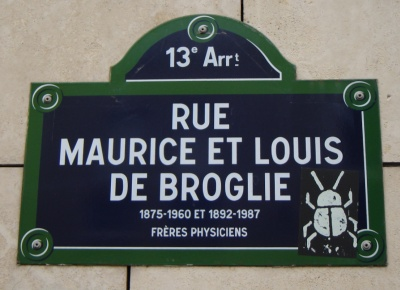Louis de Broglie won the Nobel prize in physics for his work on the wave nature of the electron, but for some reason he’s listed in the MacTutor biographies of mathematicians and (more importantly) the list of mathematicians with Paris streets named after them. So, I decided to give him the benefit of the doubt. His brother Maurice was an experimental physicist who worked on X-ray diffraction and spectroscopy.

It’s sort of amazing that Louis lived until 1987! One thinks of him as one of the early figures in quantum mechanics.

Posted by: John Baez on July 10, 2007 5:27 PM | Permalink | Reply to this

Louis de Broglie and Bohmian mechanics

John mentioned Louis de Broglie… which reminds me of one of my toy fascinations, Bohmian mechanics. Recall that David Bohm’s particle-friendly interpretation of quantum mechanics was an extension of de Broglie’s “pilot wave” ideas in the 1920’s.

I’m only interested here in the simple, purely mathematical fact which underlies Bohmian mechanics; we leave aside any philosophical and meta-philsophical “spin”.

I’m wondering if there is anybody out there who has also thought a bit about this stuff (Urs? John?) and could help me with some of the finer points.

So let’s start. For simplicity, and definiteness, we’re interested in the quantum mechanical time evolution of 1d wave functions, according to the Schrodinger equation

(1)$\frac{\partial \psi(x,t)}{\partial t} = -\frac{\partial^2 \psi}{\partial x^2} + V(x) \psi.$

We notice that the Schrodinger equation has a lagrangian formulation. The relevant action is invariant under $U(1)$, so by Noether’s theorem there is an associated current

(2)$j(x,t) = Im [\psi^*(x,t) \frac{\partial}{\partial x} \psi(x,t)],$

and we have the following conservation equation:

(3)$\frac{\partial}{\partial t} | \psi(x,t) |^2 = - \frac{\partial}{\partial x} j(x,t).$

Bohmian mechanics is nothing but the simple observation that

The flow lines of the vector field $j$ can be interpreted as the trajectories of particles.

That is, suppose we start with an initial wave function $\psi_0(x) = \psi(x, 0)$, and evolve it according to the Schrodinger equation to form $\psi(x,t)$ and hence also $j(x,t)$.

Let $a(t), b(t)$ be the flow-lines of particles initially located at $a, b$ on the $x$-axis at time $t=0$, which evolve according to the current $j$:

(4)$\frac{da}{dt} = j, \frac{db}{dt} = j.$

Then the conservation equation tells us that the probability of finding the particle between $a(t)$ and $b(t)$ remains constant as the flow proceeds:

(5)$\int_{a(t)}^{b(t)} |\psi(x,t)|^2 = \int_{a}^{b} |\psi(x,0)|^2.$

Said more elegantly, one can integrate the current $j(x,t)$ to obtain a one-parameter group of diffeomorphisms

(6)$\phi_t : \mathbb{R} \rightarrow \mathbb{R}, \quad \phi_0 = id$

and as long as we work in this “moving frame”, there is no probability change at all!

In other words, mathematically speaking, the time evolution of wavefunctions can be thought of either as literally the evolution, in time, of a wavefunction (sic!), or else as a smooth family of diffeomorphisms of space, the flowlines of which can be thought of as the trajectories of particles.

But there are some things I don’t understand. You’ll notice that the probability current $j$ vanishes if $\psi$ vanishes. Naively, this would imply that the zero’s of a wavefunction must remain stationary as the flow proceeds. How do they move?

Posted by: Bruce Bartlett on July 13, 2007 1:44 AM | Permalink | Reply to this

Re: Louis de Broglie and Bohmian mechanics

I’m wondering if there is anybody out there who has also thought a bit about this stuff (Urs? John?) and could help me with some of the finer points.

Once, in the good old days of the newsgroup sci.physics.research, I was very interested in all questions like this, concerning the relation between quantum mechanics and stochastical mechanics.

Much time has passed since. But Google remembers.

Maybe the most useful thing I did back then was writing a summary of the ideas of Edward Nelson on how complex quantum mechanics is secretly a theory of classical stochastic processes. Unfortunately, this dates back to a time where I had not yet adopted the habit of writing everything in the universally accepted code: meaning, it’s written in a funny exotic language called German:

Überblick zur stochastischen Interpretation der Quantenmechanik

But there are some things I don’t understand. You’ll notice that the probability current $j$ vanishes if $\psi$ vanishes. Naively, this would imply that the zero’s of a wavefunction must remain stationary as the flow proceeds. How do they move?

Such “nodal points”, as I think they are being called, are a major issue in these stochastic interpretations. At the end of the notes linked to above I review some literature which talks about that. (But I would have to reread this stuff myself in order to refresh my memory!)

There were even attempts to explain things like the cloud pattern on Jupiter by translating a stochastic process supposedly modelling it into the corresponding (mathematically equivalent) Schrödinger equation. But that probably goes beyond established fact.

At some point my interest moved on to other questions. I feel inclined to but do hesitate to say that the urge to understand quantum mechanics on the grounds of stochastical mechanics (over and above the mere idea of “Wick rotation”) is a sin of the idealistic youth.

On the other hand, minds greater than mine have put considerable thought into this. Most notably maybe t’Hooft. I haven’t followed this, but in Talking to ‘t Hooft about tossing TVs I recount a short conversation over dinner I had with him once on this topic.

At that entry also a couple of other interesting links are mentioned. For instance Stephan Adler from IAS has thought about this. But I never looked into his ideas.

Edward Nelson’s original rewriting of the complex Schrödinger equation as a real stochastic process of two variables was once taken up by Lee Smolin in one of his attempts to explain everything.

At one point I was very excited about all these attempts. That excitement has ceased somewhat.

I think the issue is this: Nelson’s (and the like) stochastic formulation of quantum mechanics does appeal (possibly) heavily to one’s naive physical intuition. But it has one striking drawback: it is mathematically way more intricate and way less elegant than ordinary complex quantum mechanics (even though it is, if not stretched too far, just an equivalent reformulation).

It doesn’t seem to follow the Dao.

Posted by: Urs Schreiber on July 21, 2007 12:10 PM | Permalink | Reply to this

Re: Louis de Broglie and Bohmian mechanics

I’m heartbroken! ;)

How can you mention relations between complex QM and stochastic processes without mentioning the third (maybe more beautiful approach which likely does follow the Dao), i.e. noncommutative geometry?

Discrete Differential Geometry on Causal Graphs

I’m pretty sure this does have an arrow theoretic formulation (since it is built on arrows!), but you know I’m not well enough equipped to flow with the Dao (even though I feel it ;)).

Posted by: Eric on July 21, 2007 2:47 PM | Permalink | Reply to this

Re: Louis de Broglie and Bohmian mechanics

I’m heartbroken! ;)

You shouldn’t. I may have a treat for you soon.

Let’s not wake up the baby too often before it has grown up sufficiently.

Posted by: Urs Schreiber on July 21, 2007 4:15 PM | Permalink | Reply to this

Re: Louis de Broglie and Bohmian mechanics

Hi Urs (and Eric),

I had a look at Überblick zur stochastischen Interpretation der Quantenmechanik, and it looks great. I should make the disclaimer that I am restricting myself strictly to the mathematical content of Bohmian mechanics. Thus I am sidestepping the physics issues; this gives me diplomatic immunity for not following the Dao :-)

I would just like to know a little bit more, mathematically, about this concept of a “flow being guided by a pilot wave satisfying a PDE”.

In other words, if we have some initial $\psi_0(x)$, and evolve it to $\psi(x,t)$ according to the Schrodinger equation, and if we define a time-dependent vector field by

(1)$j(x,t) = Im[\psi^* \frac{\partial}{\partial x} \psi]$

(here you can substitute your favourite Lagrangian system/conserved current instead of the Schrodinger equation), then what are the mathematical properties induced by the associated diffeomorphisms

(2)$\gamma_t : M \rightarrow M$

given by integrating $j$? (Here $M$ is space). Are they well-defined smooth isotopies of $M$? Do they exist for all time? What dynamical properties do they have? Can they give us any smooth invariants of $M$? In other words, apparantly Noether’s theorem/Bohmian mechanics is a machine which takes smooth valued initial wavefunctions,

(3)$\psi_0 : M \rightarrow \mathbb{C}$

and outputs smooth time-dependent diffeomorphisms of $M$,

(4)$\psi_0 \mapsto \gamma_t : M \rightarrow M.$

Are these diffeomorphisms mathematically interesting?

On a second point, one of the reasons I’ve always been a bit fond of this kind of thing, is because it provides, at least in principle, a way to write down the “exact solution” of a quantum field theory, in the Schrodinger picture.

The way I understand it (e.g. Hatfield), in QFT we are trying to solve for the time evolution of the wave functional,

(5)$\frac{\delta \Psi}{\delta t} = H \Psi$

where

(6)$\Psi(\cdot) : Fields(M) \rightarrow \mathbb{C}$

is the wave functional (it eats a field on $M$ and spits out a number).

Now in my understanding it is very difficult to write down a general wavefunctional

(7)$\Psi = \Psi(\phi(x), t)$

(of course that notation is not relativistically invariant, let’s ignore this for now!). The best you can do is to break it down into modes, and write the result as a huge series.

But the Bohmian picture says that, at least mathematically, the time evolution of the single wavefunctional $\Psi$ is equivalent to a time-dependent flow on the space of fields,

(8)$\gamma_t : Fields(M) \rightarrow Fields(M)$

There is the same information content in either picture; it’s just that the latter is conceptually easier to write down… though I admit that this flow on the space of fields will be non-local.

Posted by: Bruce Bartlett on July 24, 2007 11:07 PM | Permalink | Reply to this

Re: Louis de Broglie and Bohmian mechanics

Hi Bruce,

that sounds like an interesting question. But I must say I am slightly confused about what exactly you have in mind when you say “Bohmian picture”.

To me, the “Bohmian picture” is that which is obtained by rewriting the complex-linear Schrödinger equation $\frac{d}{d t} \psi(x,t) = \nabla^2\psi(x,t) + V \psi(x,t)$ as two coupled real differential equations, one of which is the continuity equation $\frac{d}{d t} \rho(x,t) = \mathrm{div} j(x,t)$ for a density $\rho$ evolving under the flow of a rotation-free vector field $j$, and the other is something like a classical Hamilton-Jacobi equation, for the potential $S$ $j = \nabla S$ of the flow, which asserts that $\frac{d}{d t} S = V + (\nabla S)^2 + Q$ up to signs and prefactors, which I certainly have wrong and/or omitted.

If we’d drop the term I called $Q$ (known as the “quantum potential”) this would be the ordinary evolution of a density of states in classical mechanics.

So in the Bohmian picture one tries to keep this classical evolution as the main starting point, and explains all quantum aspects by the presence of that term $Q$.

And this turns out to be a funny term, because it depends in turn on the density $\rho$!

So a quantum system is like a funny ensemble of classical systems which do interact in a strange way.

Maybe amazingly, this last sentence can be made precise. That’s what Edward Nelson did. He described the classical stochasics processes which behave as if subject to this funny quantum potential.

Now, I am not sure: are you really concerned with this quantum potential, or maybe rather in the maybe slightly more general observation that we may re-encode complex valued wave functions $\psi$ in terms of their density $\rho$ and probability current $j$?

Posted by: Urs Schreiber on July 25, 2007 6:33 PM | Permalink | Reply to this

Re: Louis de Broglie and Bohmian mechanics

US: So a quantum system is like a funny ensemble of classical systems which do interact in a strange way.

Indeed, a very strange way. For example, as one can easily check, the ensemble of nonrelativistic hydrogen atoms in the ground state consists of electrons that stand still anywhere they like.

Some years ago, I studied the stuff intensively for a few months to see what is going on, and even wrote an arxiv article about Bohmian mechanics. My conclusions are wholly negative.

JB: If you take the space of rays in a Hilbert space, you get a symplectic manifold. […] So, quantum mechanics is a special case of classical mechanics. I can imagine fans of hidden variables liking this.

This has been known for a long time, and has been repeatedly rediscovered. If there is interest, I can probably retrieve some references.

JB: So, I keep hoping this is what the Bohmians are trying to say.

No, they don’t. They add particles with a very strange dynamics.

I still think that there may be an underlying classical picture for quantum mechanics (see the hints here), but it is definitely not Bohmian mechanics.

Posted by: Arnold Neumaier on November 6, 2009 4:46 PM | Permalink | Reply to this

Re: Louis de Broglie and Bohmian mechanics

Arnold wrote:

This has been known for a long time, and has been repeatedly rediscovered. If there is interest, I can probably retrieve some references.

A nice recent reference is the paper by Geometrical formulation of quantum mechanics by Ashtekar and Schilling. An even more recent one is The geometry of quantum mechanics by Isidro. These have some references to older work…

Posted by: John Baez on November 6, 2009 5:45 PM | Permalink | Reply to this

Re: Louis de Broglie and Bohmian mechanics

Bruce Bartlett writes:

Thus I am sidestepping the physics issues; this gives me diplomatic immunity for not following the Dao :-)

Even if you want to ignore the dao of physics, you should still follow the dao of mathematics!

However, you seem to be doing your best to do that, so I can’t complain.

I don’t understand Bohmian mechanics. But, I would if it were secretly saying that quantum mechanics is a special case of classical mechanics. Just take the classical phase space to be the space of quantum states!

A quantum state is really a ray in Hilbert space. If you take the space of rays in a Hilbert space, you get a symplectic manifold. You can think of this as a classical phase space! Any quantum Hamiltonian gives a classical Hamiltonian on this phase space, by taking expectation values. And, if you go ahead and write down Hamilton’s equations, you get precisely Schrödinger’s equation!

All this is explained here:

• Abhay Ashtekar and Troy Schilling, Geometrical formulation of quantum mechanics.

Abstract: States of a quantum mechanical system are represented by rays in a complex Hilbert space. The space of rays has, naturally, the structure of a Kähler manifold. This leads to a geometrical formulation of the postulates of quantum mechanics which, although equivalent to the standard algebraic formulation, has a very different appearance. In particular, states are now represented by points of a symplectic manifold (which happens to have, in addition, a compatible Riemannian metric), observables are represented by certain real-valued functions on this space and the Schrödinger evolution is captured by the symplectic flow generated by a Hamiltonian function. There is thus a remarkable similarity with the standard symplectic formulation of classical mechanics. Features—such as uncertainties and state vector reductions—which are specific to quantum mechanics can also be formulated geometrically but now refer to the Riemannian metric—a structure which is absent in classical mechanics. The geometrical formulation sheds considerable light on a number of issues such as the second quantization procedure, the role of coherent states in semi-classical considerations and the WKB approximation. More importantly, it suggests generalizations of quantum mechanics. The simplest among these are equivalent to the dynamical generalizations that have appeared in the literature. The geometrical reformulation provides a unified framework to discuss these and to correct a misconception. Finally, it also suggests directions in which more radical generalizations may be found.

So, quantum mechanics is a special case of classical mechanics. I can imagine fans of hidden variables liking this. So, I keep hoping this is what the Bohmians are trying to say.

But, if they were trying to say something that simple, I imagine they’d have said it by now.

By the way, there’s a lot more one can do along the lines of Ashtekar and Schilling’s paper, and I have a paper half-written that does some of this.

Posted by: John Baez on July 25, 2007 9:29 AM | Permalink | Reply to this

Re: Louis de Broglie and Bohmian mechanics

Thanks John, for pointing out this paper. I am most intrigued by this elegant geometrical language. This seems to mesh well with the kind of geometrical ideas found in eg. Brylinski.

Posted by: Bruce Bartlett on July 25, 2007 2:38 PM | Permalink | Reply to this

Re: Louis de Broglie and Bohmian mechanics

Even if you want to ignore the dao of physics, you should still follow the dao of mathematics!

However, you seem to be doing your best to do that, so I can’t complain.

I should maybe clarify: what I said was that finding classical stochastic processes which are equivalent to complex-linear quantum mechanics is possible, but seems to go against the grain of the principle of mathematical elegance.

That’s quite different from, for instance,

- Wick rotation (analytic continuation)

- or regarding Hilbert spaces as symplectic spaces

- or other approaches

which somehow re-express the QM formalism in terms of gadgets known from classical mechanics textbooks. All these approaches, to my mind at least, are mathematically elegant. However, they don’t seem to help with the philosophical problem, if you wish, that stochastic models of QM were cooked up for, and which Bohmian mechanics was supposed to help illuminate.

Posted by: Urs Schreiber on July 25, 2007 6:00 PM | Permalink | Reply to this

Re: Mathematical Paris

There’s a “Via Mascheroni” in Milan….

Posted by: onymous on July 13, 2007 6:51 AM | Permalink | Reply to this

Re: Mathematical Paris

Second is first, Third is second… and Fifth is sixth.. ?
It seems pretty clear from this ranking that First Street is the most common street in the U.S. While many First Streets have another name, without a First Street there should be no Second Street. (I am just learning the Principle of Mathematical Induction)

Posted by: tdstephens3 on July 13, 2007 1:48 PM | Permalink | Reply to this
Weblog: The n-Category Café
Excerpt: A tour of L'Observatoire de Paris.
Tracked: July 18, 2007 4:02 PM

Re: Mathematical Paris

hum… the flier about “école d’été” is not at all a mathematical summer school …

Posted by: ric on July 20, 2007 10:33 AM | Permalink | Reply to this

Re: Mathematical Paris

Paris is filled with streets and places honoring mathematicians. Don’t overlook the quite large rue Lagrange. Then there is the rue DaGuerre, a major axis of Montparnasse. Royer-Collard is a minor figure, but there is a little street for him also. On the other hand the Place du Chatelet (probably not named after the mathematician) does suggest a reference to Emilee du Chatelet.
There is the rue Monge; streets and places named for Euler;Newton;Cauchy;Galois…the list goes on and on. What you should do is get one of those pocket sized street directory books when you’re in Paris, and search for the names of your favorite mathematicians. There is even a rue Viete!

Posted by: Roy Lisker on August 7, 2008 3:39 PM | Permalink | Reply to this

Post a New Comment